# Mechanical Engineering - Strength of Materials

Exercise : Strength of Materials - Section 7
6.
The springs in cars are used to store strain energy.
Correct
Incorrect
Explanation:
No answer description is available. Let's discuss.

7.
In a stress-strain diagram for mild steel, as shown in the below figure, the point A represents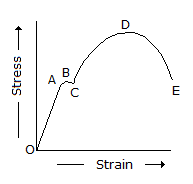elastic limit
upper yield-point
lower yield point
breaking point
Explanation:
No answer description is available. Let's discuss.

8.
When the retained material is subjected to some superimposed or surcharged load, the total horizontal pressure due to surcharged load is (where p = Intensity of the supercharged load)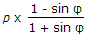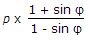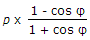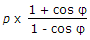Explanation:
No answer description is available. Let's discuss.

9.
The unit of Young's modulus is same as that of stress.
True
False